This book is archived and will be removed July 6, 2022. Please use the updated version.

Building Blocks of Managerial Accounting

# 10 Estimate a Variable and Fixed Cost Equation and Predict Future Costs

Sometimes, a business will need to use cost estimation techniques, particularly in the case of mixed costs, so that they can separate the fixed and variable components, since only the variable components change in the short run. Estimation is also useful for using current data to predict the effects of future changes in production on total costs. Three estimation techniques that can be used include the scatter graph, the high-low method, and regression analysis. Here we will demonstrate the scatter graph and the high-low methods (you will learn the regression analysis technique in advanced managerial accounting courses.

### Functions of Cost Equations

The cost equation is a linear equation that takes into consideration total fixed costs, the fixed component of mixed costs, and variable cost per unit. Cost equations can use past data to determine patterns of past costs that can then project future costs, or they can use estimated or expected future data to estimate future costs. Recall the mixed cost equation: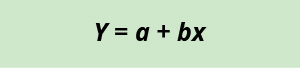where Y is the total mixed cost, a is the fixed cost, b is the variable cost per unit, and x is the level of activity.

Let’s take a more in-depth look at the cost equation by examining the costs incurred by Eagle Electronics in the manufacture of home security systems, as shown in (Figure).

Cost Information for Eagle Electronics
Cost Incurred Fixed or Variable Cost
Lease on manufacturing equipment Fixed ?50,000 per year
Supervisor salary Fixed ?75,000 per year
Direct materials Variable ?50 per unit
Direct labor Variable ?20 per unit

By applying the cost equation, Eagle Electronics can predict its costs at any level of activity (x) as follows:

1. Determine total fixed costs: ?50,000 + ?75,000 = ?125,000
2. Determine variable costs per unit: ?50 + ?20 = ?70
3. Complete the cost equation: Y = ?125,000 + ?70x

Using this equation, Eagle Electronics can now predict its total costs (Y) for any given level of activity (x), as in (Figure):

Total Cost Estimation for Various Production Levels. (attribution: Copyright Rice University, OpenStax, under CC BY-NC-SA 4.0 license)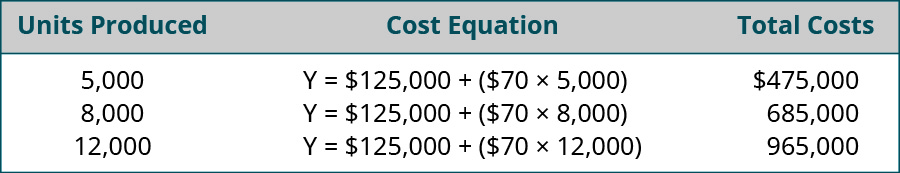When using this approach, Eagle Electronics must be certain that it is only predicting costs for its relevant range. For example, if they must hire a second supervisor in order to produce 12,000 units, they must go back and adjust the total fixed costs used in the equation. Likewise, if variable costs per unit change, these must also be adjusted.

This same approach can be used to predict costs for service and merchandising firms, as shown by examining the costs incurred by J&L Accounting to prepare a corporate income tax return, shown in (Figure).

Cost Information for J&L Accounting
Cost Incurred Fixed or Variable Cost
Building rent Fixed ?1,000 per month
Direct labor (for CPAs) Variable ?250 per tax return
Secretarial staff Fixed ?2,000 per month
Accounting clerks Variable ?100 per return

J&L wants to predict their total costs if they complete 25 corporate tax returns in the month of February.

1. Determine total fixed costs: ?1,000 + ?2,000 = ?3,000
2. Determine variable costs per tax return: ?250 + ?100 = ?350
3. Complete the cost equation: Y = ?3,000 + ?350x

Using this equation, J&L can now predict its total costs (Y) for the month of February when they anticipate preparing 25 corporate tax returns:

$$\begin{array}{c}Y=\text{?}3,000+\left(\text{?}350\phantom{\rule{0.2em}{0ex}}×\phantom{\rule{0.2em}{0ex}}25\right)\hfill \\ Y=\text{?}3,000+\text{?}8,750\hfill \\ Y=\text{?}11,750\hfill \end{array}$$

J&L can now use this predicted total cost figure of ?11,750 to make decisions regarding how much to charge clients or how much cash they need to cover expenses. Again, J&L must be careful to try not to predict costs outside of the relevant range without adjusting the corresponding total cost components.

J&L can make predictions for their costs because they have the data they need, but what happens when a business wants to estimate total costs but has not collected data regarding per-unit costs? This is the case for the managers at the Beach Inn, a small hotel on the coast of South Carolina. They know what their costs were for June, but now they want to predict their costs for July. They have gathered the information in (Figure).

Monthly Total Cost Detail for Beach Inn. (attribution: Copyright Rice University, OpenStax, under CC BY-NC-SA 4.0 license)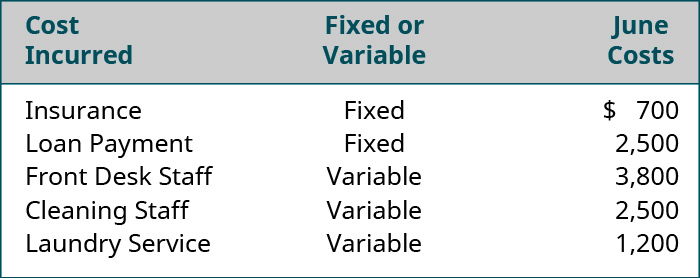In June, they had an occupancy of 75 nights. For the Beach Inn, occupancy (rooms rented) is the cost driver. Since they know what is driving their costs, they can determine their per-unit variable costs in order to forecast future costs:

$$\begin{array}{ccc}\hfill \frac{\text{Front Desk Staff}}{\text{75 nights}}& =\hfill & \frac{?3,800}{75}=?50.67\phantom{\rule{0.2em}{0ex}}\text{variable front desk staff costs per night}\hfill \\ \\ \hfill \frac{\text{Cleaning Staff}}{\text{75 nights}}& =\hfill & \frac{?2,500}{75}=?33.33\phantom{\rule{0.2em}{0ex}}\text{variable cleaning staff costs per night}\hfill \\ \\ \hfill \frac{\text{Laundry Service}}{\text{75 nights}}& =\hfill & \frac{?1,200}{75}=?16.00\phantom{\rule{0.2em}{0ex}}\text{variable laundry service costs per night}\hfill \end{array}$$

Now, the Beach Inn can apply the cost equation in order to forecast total costs for any number of nights, within the relevant range.

1. Determine total fixed costs: ?700 + ?2,500 = ?3,200
2. Determine variable costs per night of occupancy: ?50.67 + ?33.33 + ?16.00 = ?100
3. Complete the cost equation: Y = ?3,200 + ?100x

Using this equation, the Beach Inn can now predict its total costs (Y) for the month of July, when they anticipate an occupancy of 93 nights.

$$\begin{array}{c}Y=\text{?}3,200+\left(\text{?}100\phantom{\rule{0.2em}{0ex}}×\phantom{\rule{0.2em}{0ex}}93\right)\hfill \\ Y=\text{?}3,200+\text{?}9,300\hfill \\ Y=\text{?}12,500\hfill \end{array}$$

In all three examples, managers used cost data they have collected to forecast future costs at various activity levels.

Waymaker Furniture

Waymaker Furniture has collected cost information from its production process and now wants to predict costs for various levels of activity. They plan to use the cost equation to formulate these predictions. Information gathered from March is presented in (Figure).

March Cost Information for Waymaker Furniture
Cost Incurred Fixed or Variable March Cost
Plant supervisor salary Fixed ?12,000 per month
Lumber (direct materials) Variable ?75,000 total
Production worker wages Variable ?11.00 per hour
Machine maintenance Variable ?5.00 per unit produced
Lease on factory Fixed ?15,000 per month

In March, Waymaker produced 1,000 units and used 2,000 hours of production labor.

Using this information and the cost equation, predict Waymaker’s total costs for the levels of production in (Figure).

Waymaker’s Levels of Production
Month Activity Level
April 1,500 units
May 2,000 units
June 2,500 units

Solution

Total Fixed Cost = ?12,000 + ?15,000 = ?27,000. Direct Materials per Unit = ?75,000 / 1,000 Units = ?75 per unit. Direct Labor per Hour = ?11.00. Machine Maintenance = ?5.00 per unit. Total Variable Cost per Unit = ?75 + ?11 + ?5 = ?91 per unit.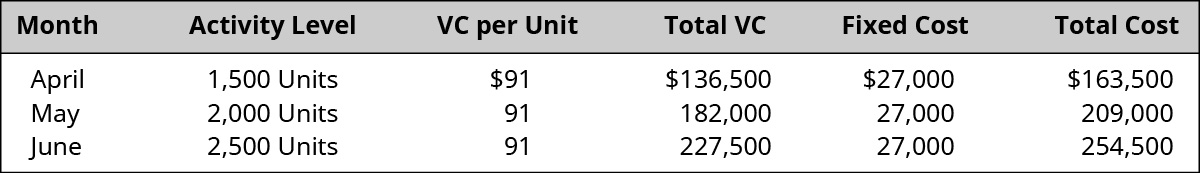### Demonstration of the Scatter Graph Method to Calculate Future Costs at Varying Activity Levels

One of the assumptions that managers must make in order to use the cost equation is that the relationship between activity and costs is linear. In other words, costs rise in direct proportion to activity. A diagnostic tool that is used to verify this assumption is a scatter graph.

A scatter graph shows plots of points that represent actual costs incurred for various levels of activity. Once the scatter graph is constructed, we draw a line (often referred to as a trend line) that appears to best fit the pattern of dots. Because the trend line is somewhat subjective, the scatter graph is often used as a preliminary tool to explore the possibility that the relationship between cost and activity is generally a linear relationship. When interpreting a scatter graph, it is important to remember that different people would likely draw different lines, which would lead to different estimations of fixed and variable costs. No one person’s line and cost estimates would necessarily be right or wrong compared to another; they would just be different. After using a scatter graph to determine whether cost and activity have a linear relationship, managers often move on to more precise processes for cost estimation, such as the high-low method or least-squares regression analysis.

To demonstrate how a company would use a scatter graph, let’s turn to the data for Regent Airlines, which operates a fleet of regional jets serving the northeast United States. The Federal Aviation Administration establishes guidelines for routine aircraft maintenance based upon the number of flight hours. As a result, Regent finds that its maintenance costs vary from month to month with the number of flight hours, as depicted in (Figure).

Monthly Maintenance Cost and Activity Detail for Regent Airlines. (attribution: Copyright Rice University, OpenStax, under CC BY-NC-SA 4.0 license)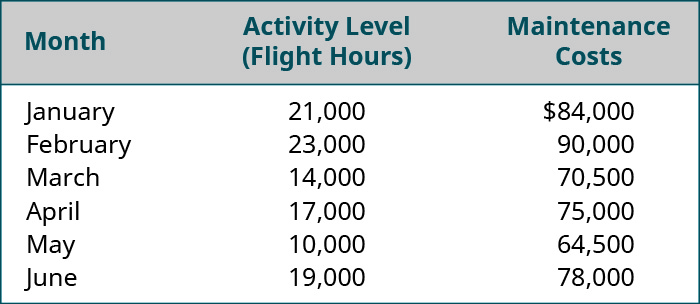When creating the scatter graph, each point will represent a pair of activity and cost values. Maintenance costs are plotted on the vertical axis (Y), while flight hours are plotted on the horizontal axis (X). For instance, one point will represent 21,000 hours and ?84,000 in costs. The next point on the graph will represent 23,000 hours and ?90,000 in costs, and so forth, until all of the pairs of data have been plotted. Finally, a trend line is added to the chart in order to assist managers in seeing if there is a positive, negative, or zero relationship between the activity level and cost. (Figure) shows a scatter graph for Regent Airlines.

Scatter Graph of Maintenance Costs for Regent Airline. (attribution: Copyright Rice University, OpenStax, under CC BY-NC-SA 4.0 license)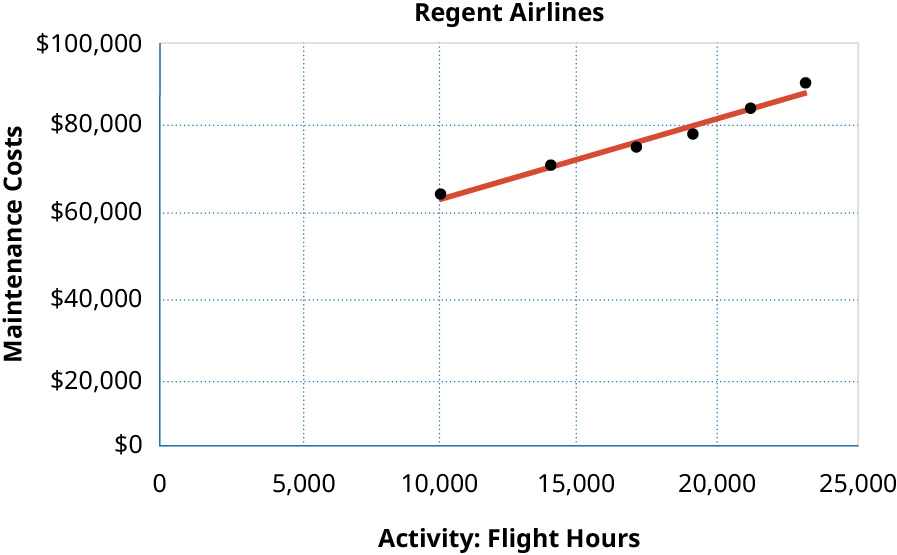In scatter graphs, cost is considered the dependent variable because cost depends upon the level of activity. The activity is considered the independent variable since it is the cause of the variation in costs. Regent’s scatter graph shows a positive relationship between flight hours and maintenance costs because, as flight hours increase, maintenance costs also increase. This is referred to as a positive linear relationship or a linear cost behavior.

Will all cost and activity relationships be linear? Only when there is a relationship between the activity and that particular cost. What if, instead, the cost of snow removal for the runways is plotted against flight hours? Suppose the snow removal costs are as listed in (Figure).

Snow Removal Costs
Month Activity Level: Flight Hours Snow Removal Costs
January 21,000 ?40,000
February 23,000 50,000
March 14,000 8,000
April 17,000 0
May 10,000 0
June 19,000 0
Scatter Graph of Snow Removal Costs for Regent Airlines. (attribution: Copyright Rice University, OpenStax, under CC BY-NC-SA 4.0 license)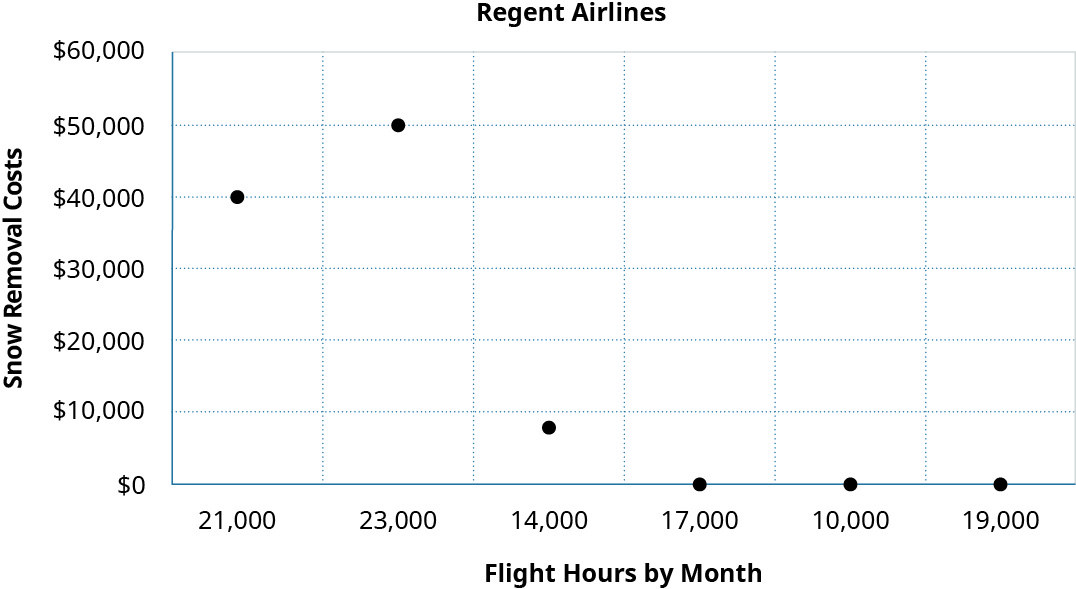As you can see from the scatter graph, there is really not a linear relationship between how many flight hours are flown and the costs of snow removal. This makes sense as snow removal costs are linked to the amount of snow and the number of flights taking off and landing but not to how many hours the planes fly.

Using a scatter graph to determine if this linear relationship exists is an essential first step in cost behavior analysis. If the scatter graph reveals a linear cost behavior, then managers can proceed with a more sophisticated analyses to separate mixed costs into their fixed and variable components. However, if this linear relationship is not present, then other methods of analysis are not appropriate. Let’s examine the cost data from Regent Airline using the high-low method.

### Demonstration of the High-Low Method to Calculate Future Costs at Varying Activity Levels

As you’ve learned, the purpose of identifying costs is to control them, and managers regularly use past costs to predict future costs. Since we know that variable costs change with the level of activity, we can conclude that there is usually a positive relationship between cost and activity: As one rises, so does the other. Ideally, this can be confirmed on a scatter graph. One of the simplest ways to analyze costs is to use the high-low method, a technique for separating the fixed and variable cost components of mixed costs. Using the highest and lowest levels of activity and their associated costs, we are able to estimate the variable cost components of mixed costs.

Once we have established that there is linear cost behavior, we can equate variable costs with the slope of the line, expressed as the rise of the line over the run. The steeper the slope of the line, the faster costs rise in response to a change in activity. Recall from the scatter graph that costs are the dependent Y variable and activity is the independent X variable. By examining the change in Y relative to the change in X, we can predict cost: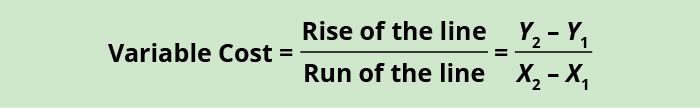where Y2 is the total cost at the highest level of activity; Y1 is the total cost at the lowest level of activity; X2 is the number of units, labor hours, etc., at the highest level of activity; and X1 is the number of units, labor hours, etc., at the lowest level of activity.

Using the maintenance cost data from Regent Airlines shown in (Figure), we will examine how this method works in practice.

Monthly Maintenance Cost and Activity Detail for Regent Airlines. (attribution: Copyright Rice University, OpenStax, under CC BY-NC-SA 4.0 license)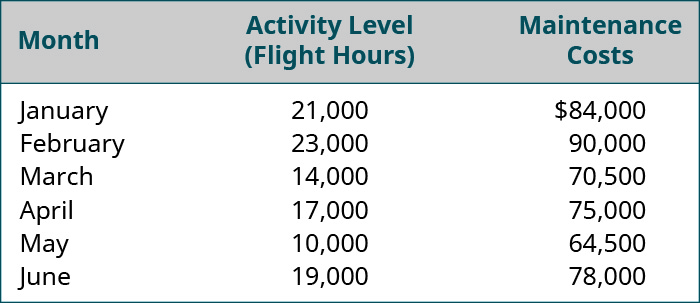The first step in analyzing mixed costs with the high-low method is to identify the periods with the highest and lowest levels of activity. In this case, it would be February and May, as shown in (Figure). We always choose the highest and lowest activity and the costs that correspond with those levels of activity, even if they are not the highest and lowest costs.

High–Low Data Points for Regent Airlines Maintenance Costs. (attribution: Copyright Rice University, OpenStax, under CC BY-NC-SA 4.0 license)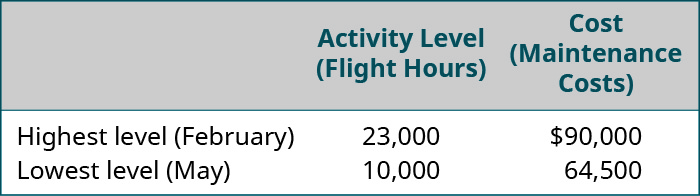We are now able to estimate the variable costs by dividing the difference between the costs of the high and the low periods by the change in activity using this formula: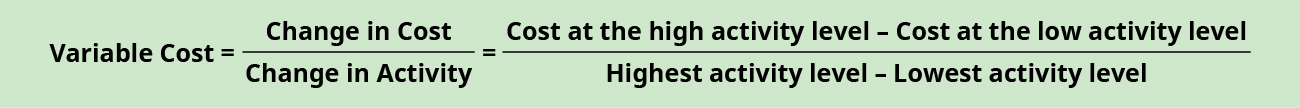For Regent Airlines, this is:

$$\text{Variable Cost}=\frac{?90,000–?64,500}{23,000–10,000}=?1.96\phantom{\rule{0.2em}{0ex}}\text{per flight hour}$$

Having determined that the variable cost per flight-hour is ?1.96, we can now determine the amount of fixed costs. We can determine these fixed costs by taking the total costs at either the high or the low level of activity and subtracting this variable component. You will recall that total cost = fixed costs + variable costs, so the fixed cost component for Regent Airlines can be isolated as shown:

$$\begin{array}{c}\text{Fixed cost}=\text{total cost}–\text{variable cost}\hfill \\ \text{Fixed cost}=?90,000–\left(23,000\phantom{\rule{0.2em}{0ex}}×\phantom{\rule{0.2em}{0ex}}?1.96\right)\hfill \\ \text{Fixed cost}=?44,920\hfill \end{array}$$

Notice that if we had chosen the other data point, the low cost and activity, we would still get the same fixed cost of ?44,920 = [?64,500 – (10,000 × ?1.96)].

Now that we have isolated both the fixed and the variable components, we can express Regent Airlines’ cost of maintenance using the total cost equation:

$$Y=\text{?}44,920+\text{?}1.96x$$

where Y is total cost and x is flight hours.

Because we confirmed that the relationship between cost and activity at Regent exhibits linear cost behavior on the scatter graph, this equation allows managers at Regent Airlines to conclude that for every one unit increase in activity, there will be a corresponding rise in variable cost of ?1.96. When put into practice, the managers at Regent Airlines can now predict their total costs at any level of activity, as shown in (Figure).

Predictions of Total Cost and Cost Components at Different Levels of Activity for Regent Airlines. (attribution: Copyright Rice University, OpenStax, under CC BY-NC-SA 4.0 license)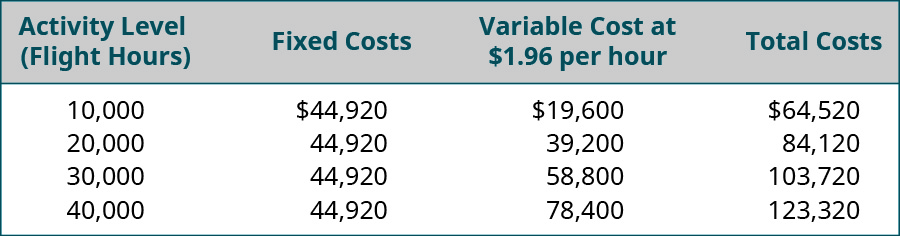Although managers frequently use this method, it is not the most accurate approach to predicting future costs because it is based on only two pieces of cost data: the highest and the lowest levels of activity. Actual costs can vary significantly from these estimates, especially when the high or low activity levels are not representative of the usual level of activity within the business. For a more accurate model, the least-squares regression method would be used to separate mixed costs into their fixed and variable components. The least-squares regression method is a statistical technique that may be used to estimate the total cost at the given level of activity based on past cost data. Least-squares regression minimizes the errors of trying to fit a line between the data points and thus fits the line more closely to all the data points.

Understanding the various labels used for costs is the first step toward using costs to evaluate business decisions. You will learn more about these various labels and how they are applied in decision-making processes as you continue your study of managerial accounting in this course.

### Key Concepts and Summary

• In order to make business decisions, managers can utilize past cost data to predict future costs employing three methods: scatter graphs, the high-low method, and least-squares regression analysis.
• Scatter graphs are used as a diagnostic tool to determine if the relationship between activity and cost is a linear relationship.
• Both the high-low method and the least-squares regression method separate mixed costs into their fixed and variable components to allow managers to predict future costs from historical costs.

(Figure)The high-low method and least-squares regression are used by managers to ________.

1. decide whether to make or buy a component part
2. minimize corporate tax liability
3. maximize output
4. estimate costs

D

(Figure)Which of the following methods of cost estimation relies on only two data points?

1. the high-low method
2. account analysis
3. least-squares regression
4. SWOT analysis.

(Figure)In the cost equation Y = a + bx, Y represents which of the following?

1. fixed costs
2. variable costs
3. total costs
4. units of production

C

(Figure)A scatter graph is used to test the assumption that the relationship between cost and activity level is ________.

1. curvilinear
2. cyclical
3. unpredictable
4. linear

(Figure)Explain how a scatter graph is used to identify and measure cost behavior.

(Figure)Explain the components of the total cost equation and describe how each of the components can be used by management for decision-making.

(Figure)Explain how the high-low method is used for cost estimation. What, if any, are the limitations of this approach to cost estimation?

(Figure)The cost data for Evencoat Paint for the year 2019 is as follows: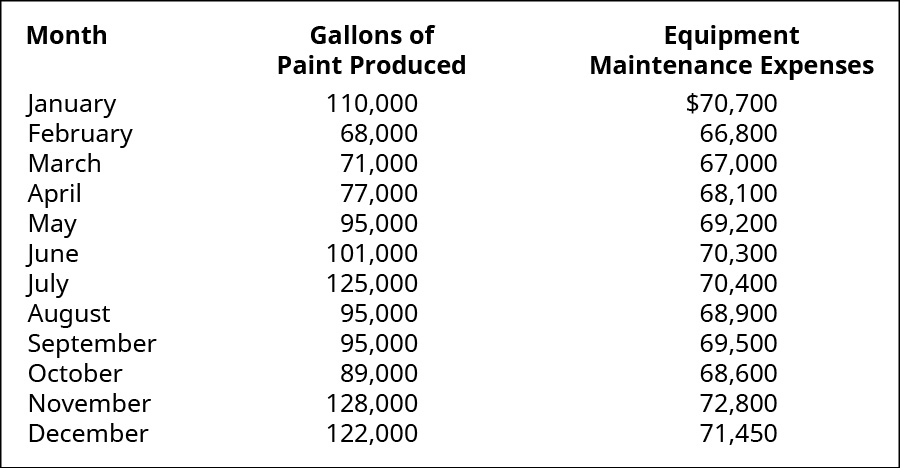1. Using the high-low method, express the company’s maintenance costs as an equation where x represents the gallons of paint produced. Then estimate the fixed and variable costs.
2. Predict the maintenance costs if 90,000 gallons of paint are produced.
3. Predict the maintenance costs if 81,000 gallons of paint are produced.
4. Using Excel, create a scatter graph of the cost data and explain the relationship between gallons of paint produced and equipment maintenance expenses.

(Figure)This cost data from Hickory Furniture is for the year 2017.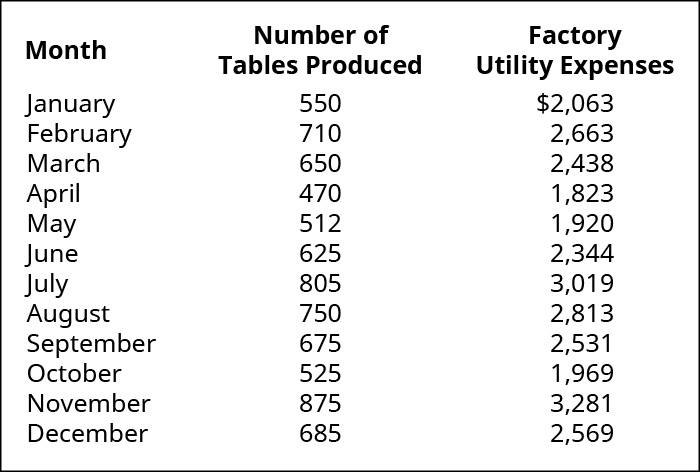1. Using the high-low method, express the company’s utility costs as an equation where X represents number of tables produced.
2. Predict the utility costs if 800 tables are produced.
3. Predict the utility costs if 600 tables are produced.
4. Using Excel, create a scatter graph of the cost data and explain the relationship between number of tables produced and utility expenses.

(Figure)Markson and Sons leases a copy machine with terms that include a fixed fee each month plus a charge for each copy made. Markson made 9,000 copies and paid a total of ?480 in January. In April, they paid ?320 for 5,000 copies. What is the variable cost per copy if Markson uses the high-low method to analyze costs?

(Figure)Markson and Sons leases a copy machine with terms that include a fixed fee each month of ?500 plus a charge for each copy made. The company uses the high-low method to analyze costs. If Markson paid ?360 for 5,000 copies and ?280 for 3,000 copies, how much would Markson pay if it made 7,500 copies?

(Figure)The cost data for BC Billing Solutions for the year 2020 is as follows: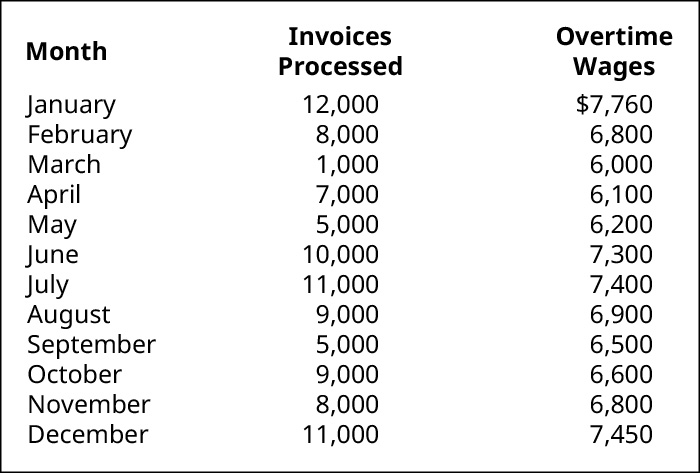1. Using the high-low method, express the company’s overtime wages as an equation where x represents number of invoices processed. Assume BC has monthly fixed costs of ?3,800.
2. Predict the overtime wages if 9,000 invoices are processed.
3. Predict the overtime wages if 6,500 invoices are processed.
4. Using Excel, create a scatter graph of the cost data and explain the relationship between the number of invoices processed and overtime wage expense.

(Figure)This cost data from Hickory Furniture is for the year 2017.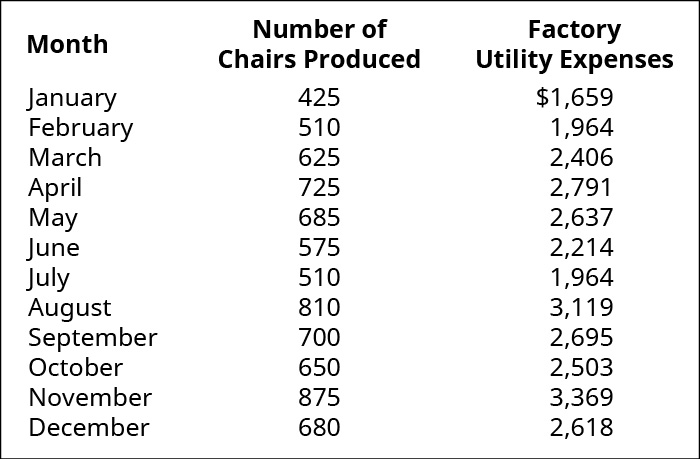1. Using the high-low method, express the factory utility expenses as an equation where x represents number of chairs produced.
2. Predict the utility costs if 900 chairs are produced.
3. Predict the utility costs if 750 chairs are produced.
4. Using Excel, create a scatter graph of the cost data and explain the relationship between number of chairs processed and utility expenses.

(Figure)Able Transport operates a tour bus that they lease with terms that involve a fixed fee each month plus a charge for each mile driven. Able Transport drove the tour bus 4,000 miles and paid a total of ?1,250 in March. In April, they paid ?970 for 3,000 miles. What is the variable cost per mile if Able Transport uses the high-low method to analyze costs?

(Figure)Able Transport operates a tour bus that they lease with terms that involve a fixed fee each month plus a charge for each mile driven. Able Transport drove the bus 7,000 miles and paid a total of ?1,360 in June. In October, Able Transport paid ?1,280 for the 5,000 miles driven. If Able Transport uses the high-low method to analyze costs, how much would Able Transport pay in December, if they drove 6,000 miles?

(Figure)Carolina Yachts builds custom yachts in its production factory in South Carolina. Once complete, these yachts must be shipped to the dealership. They have collected this shipping cost data: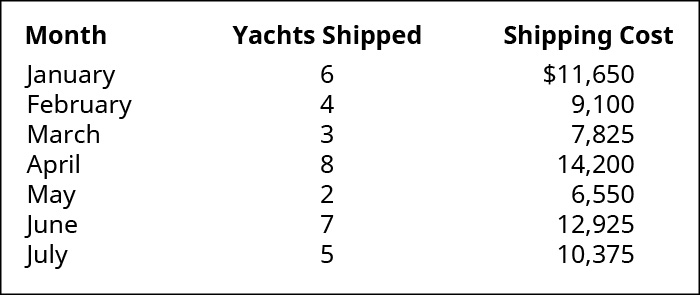1. Prepare a scatter graph of the shipping data. Plot cost on the vertical axis and yachts shipped on the horizontal axis. Is the relationship between shipping costs and unit shipped approximately linear? Draw a straight line through the scatter graph.
2. Using the high-low method, create the cost formula for Carolina Yachts’ shipping costs.
3. The least-squares regression method was used and the analysis resulted in this cost equation: Y = 4,000 + 1,275x. Comment on the accuracy of your high-low method estimation.
4. What would you estimate shipping costs to be if Carolina Yachts shipped 10 yachts in a single month? Use the cost formula you obtained in part B. Comment on how accurately this is reflected by the scatter graph you constructed.
5. What factors other than number of yachts shipped do you think could affect Carolina Yachts’ shipping expense? Explain.

(Figure)Gadell Farms produces venison sausage that is distributed to grocery stores throughout the Southeast. They have collected this shipping cost data: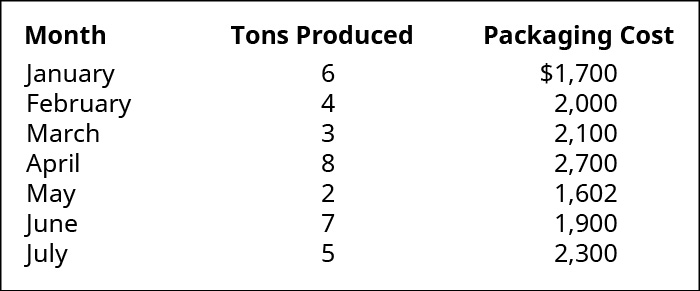1. Prepare a scatter graph of the shipping data. Plot cost on the vertical axis and tons produced on the horizontal axis. Is the relationship between packaging costs and tons produced approximately linear? Draw a straight line through the scatter graph.
2. Using the high-low method, estimate the cost formula for Gadell Farms’ packaging costs.
3. The least-squares regression method was used and the analysis resulted in this cost equation: Y = 1650 + 78.57x. Comment on the accuracy of your high-low method estimation.
4. What would you estimate packaging costs to be if Gadell Farms shipped 10 tons in a single month? Use the cost formula you obtained in part B. Comment on how accurately this is reflected by the scatter graph you constructed.
5. What factors other than number of tons produced do you think could affect Gadell Farm’s packaging expense? Explain.

### Glossary

high-low method
technique for separating the fixed and variable cost components of mixed costs
scatter graph
plot of pairs of numerical data that represents actual costs incurred for various levels of activity, with one variable on each axis, used to determine whether there is a relationship between them